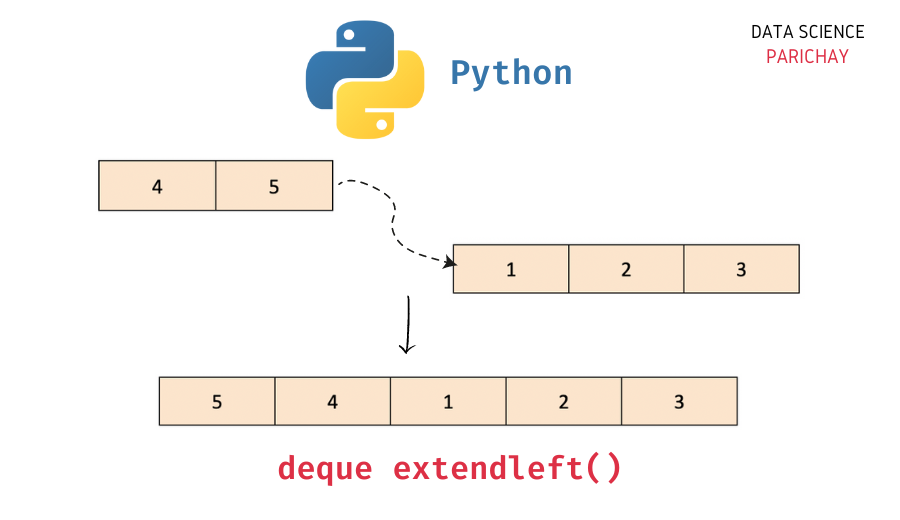# Python – Extend deque to the left

In this tutorial, we will look at how to extend a deque to the left in Python with the help of some examples.

## `deque` in Python

The `collections` module in Python comes with a `deque` class that you can use to implement a stack or queue data structure in Python. Deques support thread-safe, memory efficient appends and pops from either side of the deque with approximately the same O(1) performance in either direction.

In simple terms, you can think of a `deque` in Python as a list-like data structure but with efficient insertion and removal of items from either end (left and right). This makes them very useful when implementing a stack or queue functionality in Python.

## How to append multiple elements to the left side of deque in Python?

The `deque` class comes with a number of built-in functions to help perform common operations (like adding and removing elements).

You can extend a deque to the left if you want to append multiple elements at once to the beginning (the left side) of a deque. To extend a deque in Python to the left (append multiple elements on the left at once), use the deque `extendleft()` function.

The following is the syntax –

```# add elements from iterable to the left of deque
queue.extend(iterable)```

It appends the elements from the iterable to the left of the deque.

## Example – Append multiple elements to a deque

A common use case for the deque `extendleft()` function is when you have to add multiple elements at once to the left side of the deque.

📚 Data Science Programs By Skill Level

Introductory

Intermediate ⭐⭐⭐

🔎 Find Data Science Programs 👨‍💻 111,889 already enrolled

Disclaimer: Data Science Parichay is reader supported. When you purchase a course through a link on this site, we may earn a small commission at no additional cost to you. Earned commissions help support this website and its team of writers.

First, let’s create a deque with some elements.

```from collections import deque

# create a deque
queue = deque([1, 2, 3])
# print the deque
print(queue)```

Output:

`deque([1, 2, 3])`

Here, we imported the `deque` class from the `collections` module and created a deque object, `queue` with three elements – 1, 2, and 3.

Now, let’s add some elements to this deque object on its left side (the start of the deque).

```# add elements 4 and 5 to the left side of the deque
queue.extendleft([4, 5])
# print the deque
print(queue)```

Output:

`deque([5, 4, 1, 2, 3])`

Here, we used the `extendleft()` function to add the elements 4 and 5 to the start of the deque `queue`. We passed the elements we wanted to add on the left side inside a list (an iterable) to the `extendleft()` function.

The order in which the elements are present in the iterable is important as the same order will be used to add the elements to the deque. Here, notice that first 4 is added to the left side and then 5.

Note that, if you would’ve used the `appendleft()` function to perform the same operation, you would’ve had to call the function each time for adding each individual item separately.

## Difference between `extendleft()` and `extend()`

Both the `extendleft()` and the `extend()` functions are used to add multiple elements at once to a deque in Python. In both functions, we pass the iterable containing the elements to be added.

The main difference between them is that the `extend()` function is used to add the elements to the end (the right side) of the deque whereas the `extendleft()` function is used to add the elements to the start (the left side) of the deque.

You might also be interested in –

•